# How do I input a linear equation?

Input your equation using the virtual keyboard and tap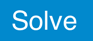to obtain the result.

You can input whole numbers, decimals, fractions, x (variable), and operation symbols.

Example 1. Solving the equation
2x - 1.5 = 3x + 2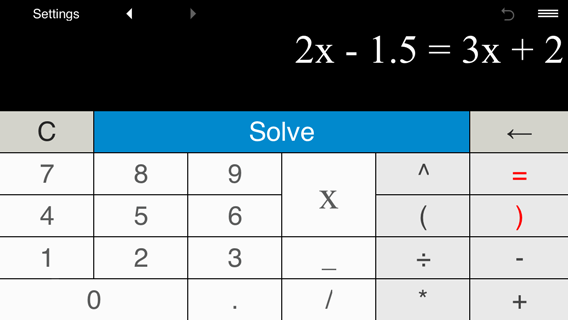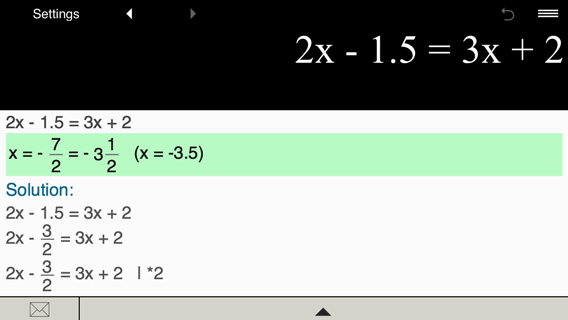Example 2. Solving the equation
1/2
x - 1
1/4
= -x - 5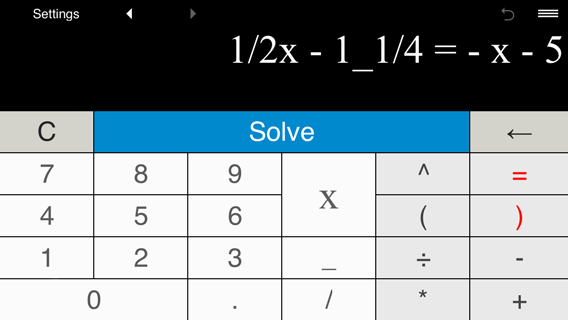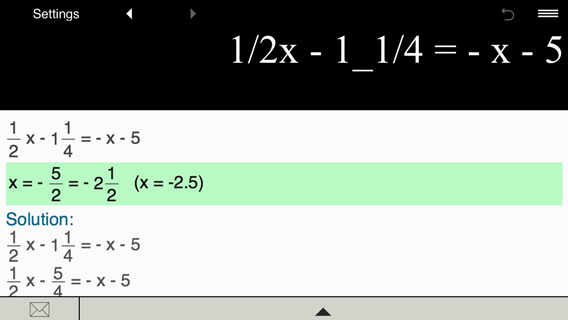Example 3. Solving the equation
5 + 3(x - 1) = 5x - 6
1/4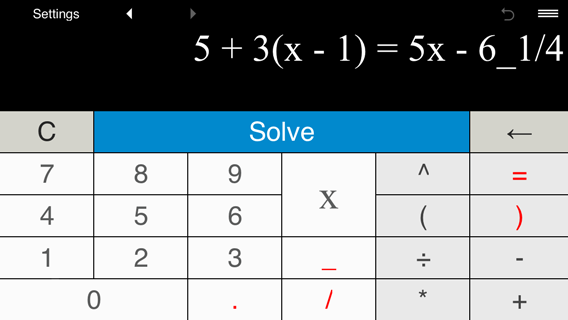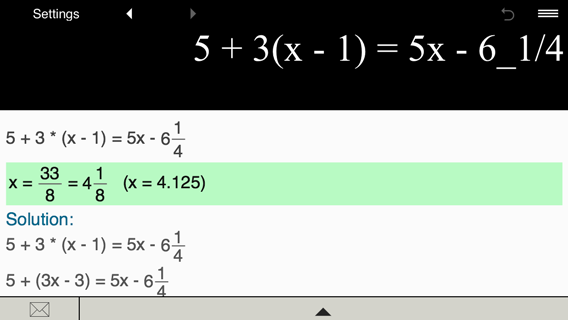Example 4. Solving the equation
2x + 3.5 =
2/5
- (4x + 5)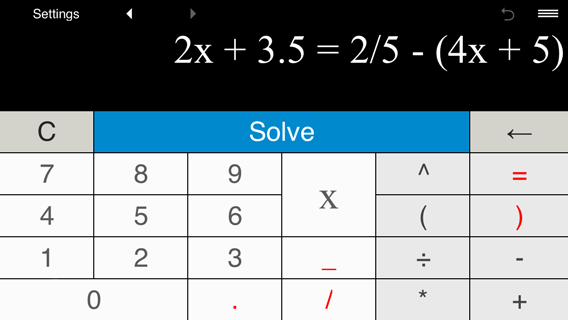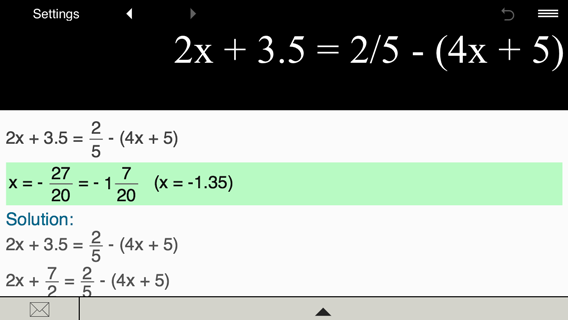Example 5. Solving the equation
(x + 1)(2x + 1) = (x + 3)(2x + 3) - 14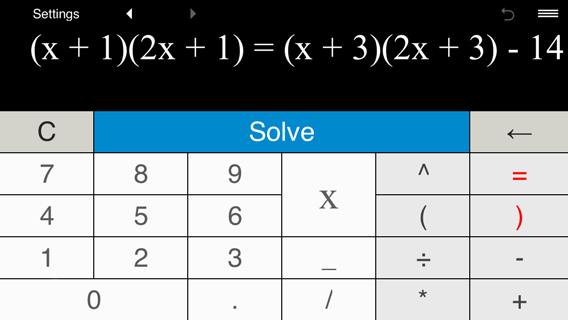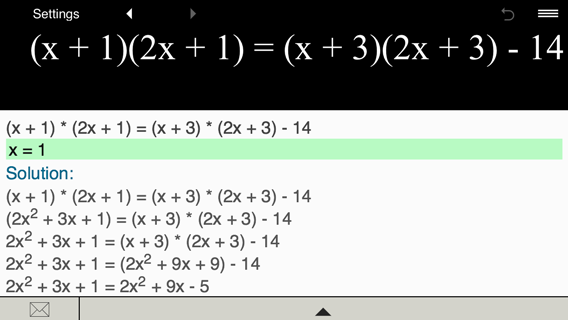Example 6. Solving the equation
3(x - 7.2) - 2(9 - 4(2 - x)) = 3
4/5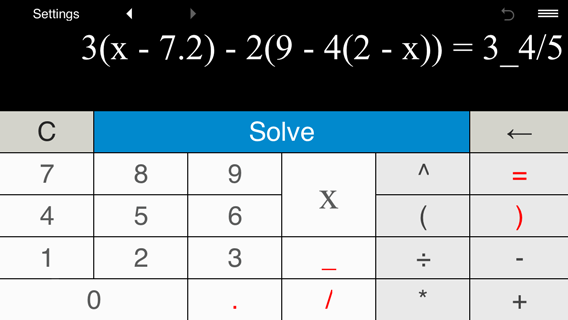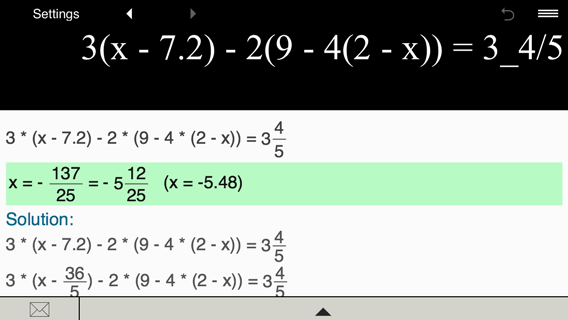Example 7. Solving the equation
(0.4x + 0.9)/0.5
=
(x - 5)/2
+ 1
1/2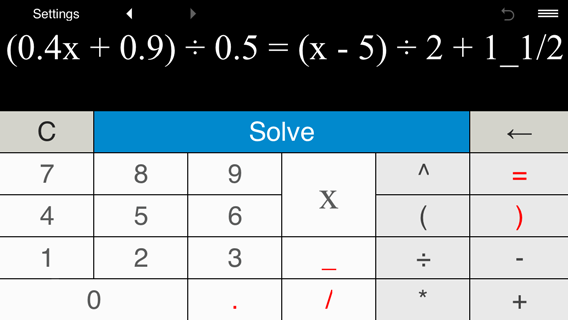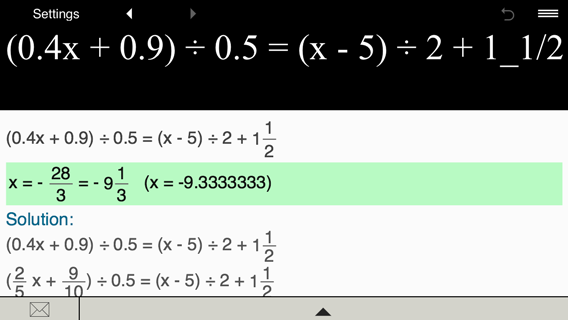Example 8. Solving the equation
3.5 = 10x - 1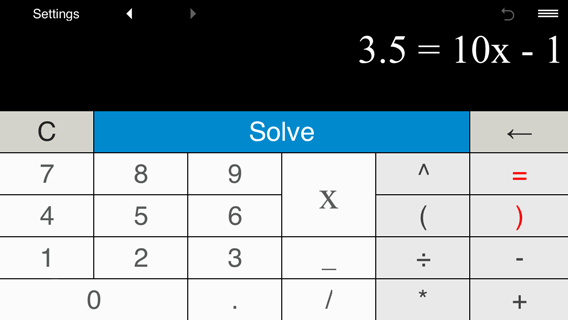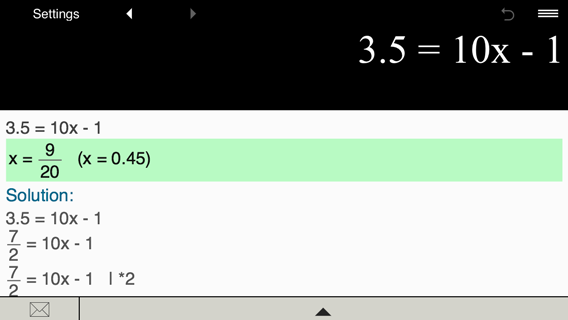Example 9. Solving the equation
4x + 5 = 3.5x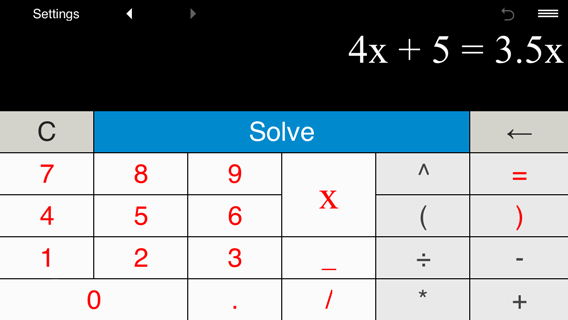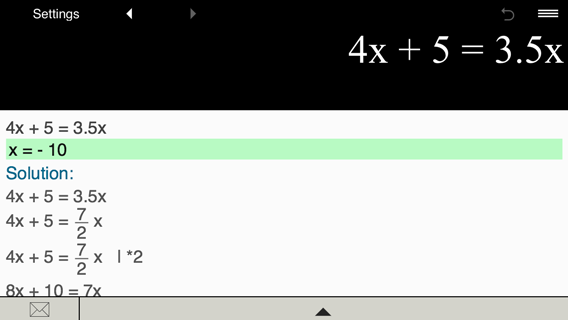Monday, December 4, 2023

Contact